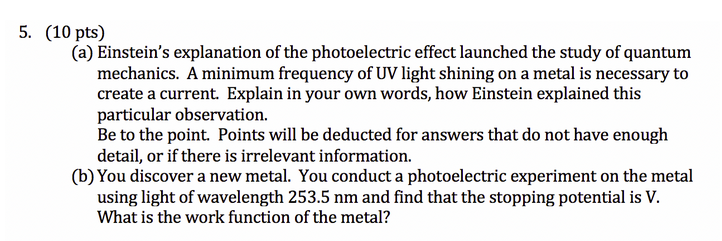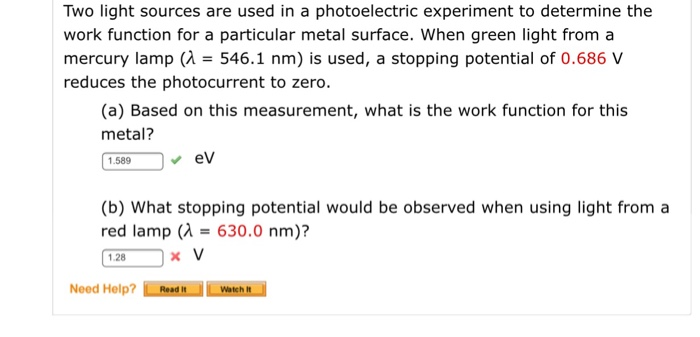QuestionThanks!

a)

A minimum frequency of UV light shining on a metal is necessary to create a current. Einstein explained this observation in the following manner.

A current can be created if free electrons are available. Each of the electron of an atom is bound to the nucleus due to Coulombian attractive force. Energy needed to be spent in order to release the electron from an atom and this can be achieved by supplying required quantity of energy in the form of UV light. The minimum energy needs to release electrons from a metal surface is called photoelectric work function. The energy of a radiation is determined by its frequency.

b)

wavelength of incident light, λ = 253.5 nm = 253.5 x 10-9 m

energy of incident light, E = hc/λ = 6.625 x 10-34 x 3 x 108 /253.5 x 10-9 m = 7.84 x 10-19 J

the stopping potential = V

kinetic energy of photoelectrons, K = eV where e is the charge of electron.

Using Einstein’s photoelectric equation,

E = W + K where W is the work function

Work function, W = E – K = E – eV = (7.84 x 10-19 – 1.6 x 10-19 V) J

Work function, W = (7.84 x 10-19 – 1.6 x 10-19 V) J

#### Earn Coins

Coins can be redeemed for fabulous gifts.

Similar Homework Help Questions
• ### Physics help! Thanks in advance! Two light sources are used in a photoelectric experiment to determine...Physics help! Thanks in advance! Two light sources are used in a photoelectric experiment to determine the work function for a particular metal surface. When green light from a mercury lamp (lambda = 546.1 nm) is used, a stopping potential of 0.686 V reduces the photocurrent to zero. (a) Based on this measurement, what is the work function for this metal? eV (b) What stopping potential would be observed when using light from a red lamp (lambda = 630.0 nm)?...